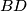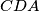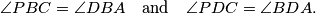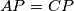### IMO Shortlist 2004 problem G4

Kvaliteta:
Avg: 0.0
Težina:
Avg: 7.0
In a convex quadrilateral$ABCD$, the diagonal$BD$ bisects neither the angle$ABC$ nor the angle$CDA$. The point$P$ lies inside$ABCD$ and satisfiesProve that$ABCD$ is a cyclic quadrilateral if and only if$AP=CP$.
Source: Međunarodna matematička olimpijada, shortlist 2004